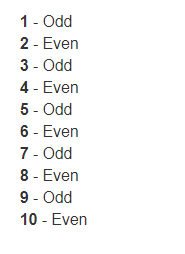# Razer View Syntax in MVC

## Razer View Syntax in MVC

In this article, we will discuss razor view syntax in the ASP.NET MVC application.

Use @ symbol to switch between C# code and HTML.

```@for (int i = 1; i <= 10; i++)
{
<b>@i</b>
}
```

Output:
1 2 3 4 5 6 7 8 9 10

Use @{ } to define a code block. If we want to define some variables and perform calculations, then use code block. The following code block defines 2 variables and computes the sum of the first 10 even and odd numbers.

```@{
int SumOfEvenNumbers = 0;
int SumOfOddNumbers = 0;

for (int i = 1; i <= 10; i++)
{
if (i % 2 == 0)
{
SumOfEvenNumbers = SumOfEvenNumbers + i;
}
else
{
SumOfOddNumbers = SumOfOddNumbers + i;
}
}
}

<h3>Sum of Even Numbers = @SumOfEvenNumbers</h3>
<h3>Sum of Odd Numbers = @SumOfOddNumbers</h3>
```

Output:
Sum of Even Numbers = 30

Sum of Odd Numbers = 25

Use <text> element or @: to switch between c# code and literal text

```@for (int i = 1; i <= 10; i++)
{
<b>@i</b>
if (i % 2 == 0)
{
<text> - Even </text>
}
else
{
<text> - Odd </text>
}
<br />
}
```
###### The above program can be re-written using @: as shown below.
```@for (int i = 1; i <= 10; i++)
{
<b>@i</b>
if (i % 2 == 0)
{
@: - Even
}
else
{
@: - Odd
}
<br />
}
```

Output:###### Use @* *@ to comment in razor views

@*This is a comment in razor views*@

The transition between c# expressions and literal text

```@{
int day = 31;
int month = 12;
int year = 2013;
}

Date is @day-@month-@year
```

Output:
Date is 31-12-2013

The @ symbol is used as a code delimiter in razor views. However, the razor is smart enough to recognize the format of internet email address and not to treat the @ symbol as a code delimiter.

This is my email address<br />

<b>info@dotnettutorials.net</b>
Use @ symbol to escape @

I will meet you @@ office

Output:
I will meet you @ office